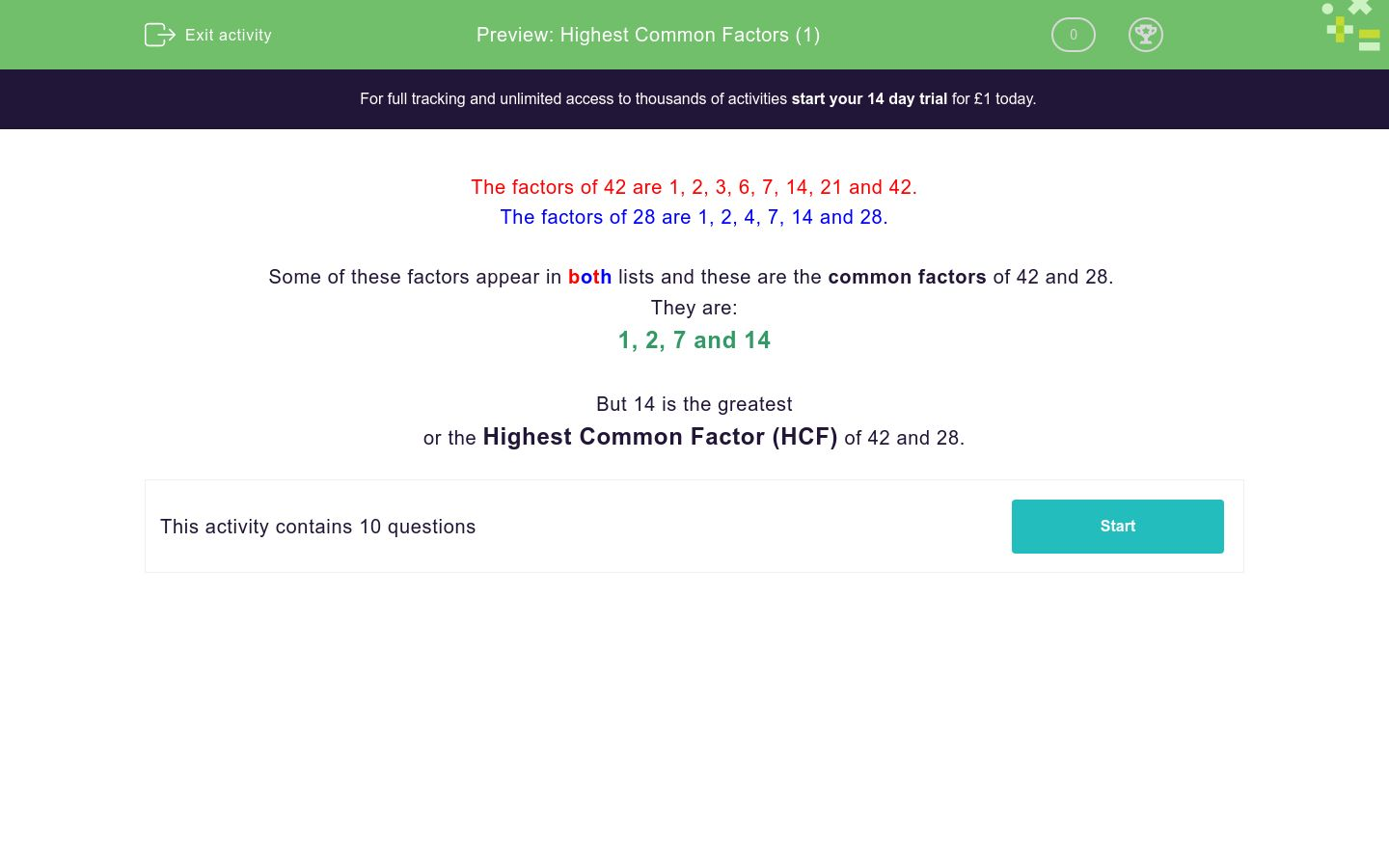# Highest Common Factors (1)

In this worksheet, students state the highest common factor of two numbers.Key stage:  KS 3

Curriculum topic:   Number

Curriculum subtopic:   Use Concepts and Vocabulary for All Numbers

Difficulty level:### QUESTION 1 of 10

The factors of 42 are 1, 2, 3, 6, 7, 14, 21 and 42.

The factors of 28 are 1, 2, 4, 7, 14 and 28.

Some of these factors appear in both lists and these are the common factors of 42 and 28.

They are:

1, 2, 7 and 14

But 14 is the greatest

or the Highest Common Factor (HCF) of 42 and 28.

What is the highest common factor of....?

2 and 5

What is the highest common factor of....?

2 and 4

What is the highest common factor of....?

3 and 4

What is the highest common factor of....?

4 and 6

What is the highest common factor of....?

3 and 6

What is the highest common factor of....?

6 and 9

What is the highest common factor of....?

14 and 21

What is the highest common factor of....?

15 and 10

What is the highest common factor of....?

24 and 36

What is the highest common factor of....?

36 and 45

• Question 1

What is the highest common factor of....?

2 and 5

1
• Question 2

What is the highest common factor of....?

2 and 4

2
• Question 3

What is the highest common factor of....?

3 and 4

1
• Question 4

What is the highest common factor of....?

4 and 6

2
• Question 5

What is the highest common factor of....?

3 and 6

3
• Question 6

What is the highest common factor of....?

6 and 9

3
• Question 7

What is the highest common factor of....?

14 and 21

7
• Question 8

What is the highest common factor of....?

15 and 10

5
• Question 9

What is the highest common factor of....?

24 and 36

12
• Question 10

What is the highest common factor of....?

36 and 45

9
---- OR ----

Sign up for a £1 trial so you can track and measure your child's progress on this activity.

### What is EdPlace?

We're your National Curriculum aligned online education content provider helping each child succeed in English, maths and science from year 1 to GCSE. With an EdPlace account you’ll be able to track and measure progress, helping each child achieve their best. We build confidence and attainment by personalising each child’s learning at a level that suits them.

Get started